# 2957. 统计不同的最简真分数的个数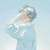### include

using namespace std;
int T,n;
int a;
struct Point
{
int xx,yy;
bool operator<(const Point& bb) const
{
if(xx!=bb.xx) return xx S;
int gcd(int aa,int bb)
{
if(aa=bb) return 0;
return 1;
}
int main()
{
std::ios::sync_with_stdio(false);
cin>>T;
for(int step=0;step>n;
S.clear();
for(int i=1;i<=n;i++) cin>>a[i];
for(int i=1;i<=n;i++)
for(int j=i+1;j<=n;j++)
{
if(ok(a[i],a[j])) S.insert((Point){a[i],a[j]});
if(ok(a[j],a[i])) S.insert((Point){a[j],a[i]});
}
printf(“case #%d:\n%d\n”,step,S.size());
}
return 0;
}

### include

using namespace std;
int T,n;
vector a,b;

int gcd(int aa,int bb)
{
if(aa=bb) return 0;
return 1;
}
int main()
{
std::ios::sync_with_stdio(false);
cin>>T;
for(int step=0;step>n;
int ans=0;
a.clear();
for(int i=1;i<=n;i++)
{
int xx;
cin>>xx;
a.push_back(xx);
}
sort(a.begin(),a.end());
b.clear();
unique_copy(a.begin(),a.end(),back_inserter(b));
for(int i=0;i<b.size();i++)
for(int j=i+1;j<b.size();j++)
{
if(ok(b[i],b[j])) ++ans;
if(ok(b[j],b[i])) ++ans;
}
printf(“case #%d:\n%d\n”,step,ans);
}
return 0;
}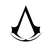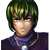#include <stdio.h>
#include <stdlib.h>
int gcd(int a, int b)
{
if(a%b) return gcd(b,a%b);
else return b;
}
int cmp(const void*a, const void*b)
{
int *p1=(int*)a, *p2=(int*)b;
return *p1-*p2;
}
int main()
{
int T, I;
scanf("%d",&T);
for(I=0; I<T; I++)
{
int n, i, j, x, num, cnt=0;
scanf("%d",&n);
for(i=0; i<n; i++)
{
scanf("%d",&num[i]);
for(j=0; j<i; j++) if(num[j]==num[i]) {n--; i--; break;}
}
qsort(num, n, sizeof(int), cmp);
for(i=0; i<n-1; i++)
for(j=i+1; j<n; j++)
{
if(!(num[i]-num[j])) continue;
x=gcd(num[j], num[i]);
if(x==1) cnt++;
}
printf("case #%d:\n%d\n",I,cnt);
}
return 0;
}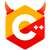#include <algorithm>
#include <iostream>
#include <numeric>
#include <set>
#include <vector>

using namespace std;

struct Fraction {
int numerator;
int denominator;
bool operator<(Fraction const &frac) const {
return numerator * frac.denominator < frac.numerator * denominator;
}
};

int main() {
ios::sync_with_stdio(false);
int t;
cin >> t;
for (int i = 0; i < t; ++i) {
int n;
cin >> n;
set<Fraction> pool;
vector<int> nums(n);
for (auto &i : nums)
cin >> i;
sort(nums.begin(), nums.end());
for (int j = 0; j < n; ++j)
for (int k = j + 1; k < n; ++k)
if (gcd(nums[k], nums[j]) == 1)
pool.insert({nums[j], nums[k]});
pool.erase({1, 1});
cout << "case #" << i << ":\n" << pool.size() << '\n';
}
}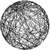#include <algorithm>
#include <iostream>
#include <numeric>
#include <set>
#include <vector>

using namespace std;

struct Fraction {
int numerator;
int denominator;
bool operator<(Fraction const &frac) const {
return numerator * frac.denominator < frac.numerator * denominator;
}
};

int main() {
ios::sync_with_stdio(false);


from math import gcd


    int t;
cin >> t;
for (int i = 0; i < t; ++i) {
int n;
cin >> n;
set<Fraction> pool;
vector<int> nums(n);
for (auto &i : nums)
cin >> i;
sort(nums.begin(), nums.end());


T = int(input())
for I in range(T):
input()
lst = list({int(a) for a in input().split()})